## Fluid Mechanics and Hydraulic Machinery Miscellaneous

#### Fluid Mechanics and Hydraulic Machinery

1. Circulation is defined as line integral of tangential component of velocity about a _______ (fill in the blanks)

1. In a flow field, closed contour exists.

##### Correct Option: C

In a flow field, closed contour exists.

1. Shown below are three pipe sections through which water flows as shown. Option a to d below pertain to the direction of the net force on the pipe section due to the flow of water.
45° to both +ve x and +ve y axes
45° to both -ve x and +ve y axes
45° to both +ve x and -ve y axes
45° to both -ve x and -ve y axes
State which of the options indicate the correct direction of force for pipe 1, pipe 2 and pipe 3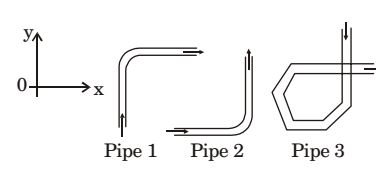1. 1 – d, 2 – d, 3 – b

##### Correct Option: A

1 – d, 2 – d, 3 – b

1. The stream function in a two dimensional flow field is given by ψ = x² – y² The magnitude of the velocity at point (1, 1) is

1. Stream function, ψ = x² – y²

 u = -∂ψ = -∂ (x2 - y2) = 2y ∂y ∂y

 or V = -∂ψ = ∂ (x2 - y2) = 2x ∂x ∂x

V = uî + vĵ at (1, 1) = 2î + 2ĵ
V = √(2)² + (2)² = 2√2

##### Correct Option: B

Stream function, ψ = x² – y²

 u = -∂ψ = -∂ (x2 - y2) = 2y ∂y ∂y

 or V = -∂ψ = ∂ (x2 - y2) = 2x ∂x ∂x

V = uî + vĵ at (1, 1) = 2î + 2ĵ
V = √(2)² + (2)² = 2√2

1. In given figure, if the pressure of gas in bulb A is 50 cm Hg vacuum and patm = 76 cm Hg, then height of column H is equal to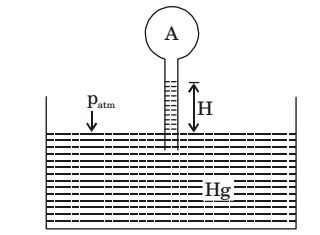1.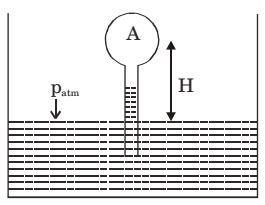pA = 50 cm Hg vacuum = pH

##### Correct Option: BpA = 50 cm Hg vacuum = pH

1. Refer to figure, the absolute pressure of gas A in the bulb is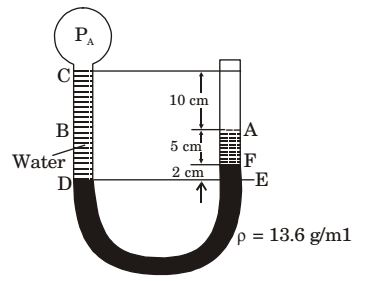1. Patm = 760 mm Hg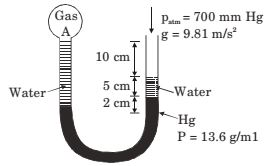Let PA be the absolute pressure at 'A'
Pressure at D = Pressure at E
or PA + PCD = Patm + PAF + PFE

 PA + 170 = 760 + 50 + 20 13.6 13.6

PA = 771.2 mm Hg

##### Correct Option: A

Patm = 760 mm HgLet PA be the absolute pressure at 'A'
Pressure at D = Pressure at E
or PA + PCD = Patm + PAF + PFE

 PA + 170 = 760 + 50 + 20 13.6 13.6

PA = 771.2 mm Hg